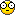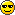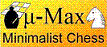## Yet another new bitboard move generation method

Programming Topics (Computer Chess) and technical aspects as test techniques, book building, program tuning etc

Moderator: Andres Valverde

### Re: Yet another new bitboard move generation method

OK, yet another one, based on a very similar idea: Quitboards...(Perhaps it should stop here?)

Generating moves, starting from the piece that makes it, is simple enough for me, even in 0x88/mailbox representations. What I'm constantly struggling with is a fast way to generate captures. And not just generate them in bulk, but generate them in specific sub-groups. Basically, I want a move generator that simulates an attack table.

So what I would like is not an algorithm that looks at the occupation of a ray and tells me where I can go on that ray, but one that looks at the occupation of a ray and tells me what can hit me from it. In particular Slider moves, since Pawn attacks are easy enough to detect from the board (can only come from 2 squares), and Leapers attacks from the piece list. (There are only 3 Leapers, and the0x88 test immediately tells you if they can capture.) It is the Slider moves, with all the ray scanning, that are a pain. Also in differentially updated attack tables: for every move you do, you have to wonder which Sliders were passing over the to-square, and which are now passing over the from-square.

To know which Sliders attack me along a given ray, I need to distinguish not 3, but 4 possible states of each square: empty, obstacle, a slider of mine that moves along this ray, and a similar slider of the opponent. All 8 squares along a ray are important, since an attack can come from an edge square. So the edge squares need at least 3 states: {my slider, his slider, other}. There might not be enough savings there to treat it special.

So my idea is to keep a 16-bit int for each rank (8), file (8) or diagonal (2 x 15). There would be a table RayNr that points you to the ray passing through a given square in a given direction. The 'board representation' itself would only need 46 shorts, so they could be pointed to by a 1-byte RayNr.

For each move you would have to update the 4 rays going through the from-square, and the 4 rays going through the to-square. (A bit similar to rotated bitboards.) To do this efficiently you could keep an array short int Masks that has the two bits set that correspond to the particular square within the ray representation.

Once you have the board representation, you can quickly get the Slider attacks to a particular square SQ by taking each of the four rays r=Ray[RayNr[SQ][i]], i=0..3, and use them as an index in a lookup table together with the position within the ray (which could come from a table RayPos, that tabulated file and rank numbers, but in practice is probably derived more quickly from masking/shifting the square number itself). This table would then tell you what was going on along this ray: from which squares does an attack come, is it a doubled attack, etc.

The latter table would have a substantial size: 64K x 8 = 512K. (Times the element size!) It could be shared by all ray types, though (othogonals and diagonals). Short diagonals would simply pad the non-existent squares with empties or obstructions, so that they would use the correct subset of the table.

In principle you would also need two different tables for opposite colors, but you can flip the color content of a ray representation easily enough by clever encoding: make the upper byte contain one bit per square that is 0 for empty or obstruction, and 1 for an active Slider. The lower byte would in the first case make the distiction between empty and obstruction, in the second case between white or black. The color is then flipped by x ^= x>>8;

The square that you land on is not important for what can hit you (or what you can hit, for that matter, the table could also contain your own moves from that square, in addition to the captures to it!) . You could clear the corresponding 2 bits, reducing the table size by a factor 4. For all but the upper bits that would not give a contiguous range, though, but I think you can solve that by using (x ^ (x>>1)) & 0x3FFF as an index. (Haven't thought about that very deeply, so I might be wrong there...)

With 16K x 8 = 128K elements the table size seems manageable, and you could just about tabulate anything you wanted (bitboards with attacker positions, but also lists of square numbers where the attackers are on. (Unlike your own moves, which could total 7, there can only be upto two captures, in 30 different layouts, so a 5-bit value to be used as an index to look up the actual squares would suffice.) The amount of work you would need to get at it would be modest. And no 64-bit stuff, everything can be done in 16-bit arithmetic.No multiplications either.H.G.Muller

Posts: 3370
Joined: 16 Nov 2005, 12:02
Location: Diemen, NL

### Re: Yet another new bitboard move generation method

To squeeze out a hole of 2 bits out of the index you would need to shift 2 places ((x ^ x>>2) & 0x3FFF), of course, even if the missing bits are scattered (non-contiguous). Otherwise the MSB would obviously not participate.

The loop over directions for updating the 'quitboard' (or actually, only its relevant rays) or interrogating it is likely to be unrolled. So it is better to use the index order char RayNr, so that RayNr[d] is translated as a constant. For my 0x88 square numbering I would of course need RayNr, and the table would have holes for the unused square numbers. I could fill up the holes, if I wanted, by interleaving with other 0x88 boards with byte-sized elements, or even interleave the orthogonals and diagonals.

Since the rays are only 16-bit in length, I could pack 2 of them in an int for more efficient updating. If I store them as ints, I could, for instance, store the file occupations in the high-order 16 bits, and the rank occupations in the low-order 16. In each case, the other half would stay empty (=0). The updating on Make() and UnMake() could then go as:

Code: Select all
`/* Piece codes */#define P_OBSTACLE 0x00FF00FF#define P_WMOVER 0xFF00FF00#define P_BMOVER 0xFFFFFFFFint PieceCode; /* contains piece codes per (piece,direction)     *//* for instance, a white Bisshop would have P_WMOVER for its diagonals  *//* (d=2,3) and P_OBSTACLE for orthogonals (d=0,1)                       */char RayNr; /* 'point to' rays in each direction for this square */int Ray;       /* occupations of 16 orthogonals and 30 diagonals    */int Mask= {   /* marks the bits used for this square in Ray for this direction */    {        0x01010101, /* a1 */        0x02020101, /* b1 */        ....        0x03032020, /* c6 */        ....'        0x80808080  /* h8 */    },    /* for d=1 would be the same as d=0 */    ...};Make(){    /* remove piece from old position */    for(d=0; d<4; d++)    {   PUSH(Ray[RayNr[d][FROM]); /* for easy UnMake */        Ray[RayNr[d][FROM]] &= ~Mask[d][FROM];    }    /* Put in new position */    for(d=0; d<4; d+=2)               /* steps of 2! */    {   int h;        h = Ray[RayNr[d][TO]] | Ray[RayNr[d+1][TO]]; /* pack */        PUSH(h);        h &= ~Mask[d][TO];                         /* erase victim */        h |= Mask[d][TO] & PieceCode[ColoredPieceType][d];        Ray[RayNr[d][TO]] = h & 0x0000FFFF;       /* write back */        Ray[RayNr[d+1][TO]] = h & 0xFFFF0000;    }}`

For consultation you would use something like:
Code: Select all
`char InternalPos; /* for files (d=1): rank number. Others, file nr. */char First, Second; /* vectors from threatened square to attacker */char Attackers[0x4000]; /* main data table */{    int h;    /* rank (d=0) */    h = Ray[RayNr[SQ]];    if(color == BLACK) h ^= h>>8; /* B/W -> mine/his */    h &= ~Mask[SQ]; /* clear target square */    h = (h>>16 ^ h>>18) & 0x3FFF;    s = Attackers[h][InternalPos[SQ]];    AttackSqr1 = SQ + First[s]; /* probably a push on an attackers stack */    AttackSqr2 = SQ + Second[s];    /* file (d=1) */    h = Ray[RayNr[SQ]];    if(color == BLACK) h ^= h>>8; /* B/W -> mine/his */    h &= ~Mask[SQ]; /* clear target square */    h = (h ^ h>>2) & 0x3FFF;    s = Attackers[h][InternalPos[SQ]];    AttackSqr3 = SQ + First[s];    AttackSqr4 = SQ + Second[s];    /* similar for diagonals and anti-diagonals */    ...}`

This is for the case were I only want to generate the slider attacks to the given square SQ. The internal position on the ray of these attackers can be encoded by a number from 0 to 29 (0 = no attackers, 1-8 = single attack from indicated internal position, and then 1+2+3+4+5+6 = 21 combinations of 2 squares with at least one square in between). The small tables First and Second would be used to translate this encoding to an actual step vector, because the mapping from internal ray position to board position depends on the ray direction. Having only 30 attacker combinations of course leaves 3 bits to store other information that might be of interest. Such as X-ray attack from left/right possible, pin/discovered threat activated (= an X-ray attack through an obstacle in stead of a same-side mover)...H.G.Muller

Posts: 3370
Joined: 16 Nov 2005, 12:02
Location: Diemen, NL

### Re: Yet another new bitboard move generation method

The íf(color==BLACK) stuff would in practice of course be done branchless, through:
Code: Select all
`int ColorMask = { 0, 0xFF00FF00 };    h ^= (h & ColorMask[color]) >> 8;`

In fact it seems possible to be smarter here: there is no need to distinguish own pieces (i.e. of the side you are not generating the moves for) from other blockers. This might not only offer simpler ways to convert the detailed ray occupation (specifying the movers for both colors) into a color-specific lookup key (where pieces of the opponent are converted to one code, independent of which color the opponent is, while my pieces are converted to obstacles). Since at the lookup stage there are only 3 states per square, it might also allow to shrink the table further.

One way to do it would be to switch back to a Ray encoding where each square is again represent by two neighboring bits. The lower and upper byte could then be translated separately from quaternary to ternary through a color-dependent table of 2 x 256 entries, filled such that the distinction between empty, obstacle and own piece for the edge square is ignored. This would leave 54 possibilities for each byte (2*3*3*3). The pair of bytes would leave 54*54 = 2916 possibilities. This would already shrink the Attackers table to less than 8 x 3K = 24K, even without making use of the whole at the internal position. That seems small enough to not worry at all about this hole. Interrogating the table would than go like:
Code: Select all
`short int MapH, MapL; /* Quaternary to ternary translation */{   unsigned short int h;    /* rank (d=0) */    h = Ray[RayNr[SQ]];    h = MapL[color][h & 0xFF] + MapH[color][h >> 8];    s = Attackers[h][InternalPos[SQ]];    AttackSqr1 = SQ + First[s]; /* probably a push on an attackers stack */     AttackSqr2 = SQ + Second[s];     /* file (d=1) */     h = Ray[RayNr[SQ]] >> 16;    h = MapL[color][h & 0xFF] + MapH[color][h >> 8];    s = Attackers[h][InternalPos[SQ]];     AttackSqr3 = SQ + First[s];     AttackSqr4 = SQ + Second[s];     /* similar for diagonals and anti-diagonals */     ... }`

A smart compiler would of course translate the bit juggling with Ray[] before using it as an index in MapX[][] (X=H,L) as a simple 8-bit access (with zero-extend) to the required two bytes of the indicated Ray, immediately using the loaded data as an index into MapX[][] without any shifting or ANDing. MapH would be filled with multiples of 54, to be directly addable to MapL without the need for a multiplication. MapH and MapL could be the same table if we are prepared to throw in an extra multiplication (by 54), and take care to map the edge square to the same bits in higher and upper byte of the Ray.H.G.Muller

Posts: 3370
Joined: 16 Nov 2005, 12:02
Location: Diemen, NL

### Re: Yet another new bitboard move generation method

This latter method could still go a long way:

since the Ray representation of the board only accesses 8 squares at a time, you can afford 4 bits of info per square. This is enough to store the exact state of the board (piece-type + color), since each square can only have 13 states in chess. The information would be stored 4 times redundantly, to make it easy to access the correct groups of 8 squares. Just as in rotated bitboard (it would actually be a rotated nybble board).

The strategy would be to collapse the full information on the squares into relevant information for the current purpose. If I am generating black attacks to a given square, all white pieces and black pieces that don't move along the ray are equivalent to obstacles. (And on an edge square that again is equivalent to empty.) This collapsing could be done on fragments of the ray (through MapX tables) per 3 or 4 squares simultaneously. The collapsed state of the ray fragments would then be combined to a full state of the ray, which could be used as index into the main lookup table.H.G.Muller

Posts: 3370
Joined: 16 Nov 2005, 12:02
Location: Diemen, NL